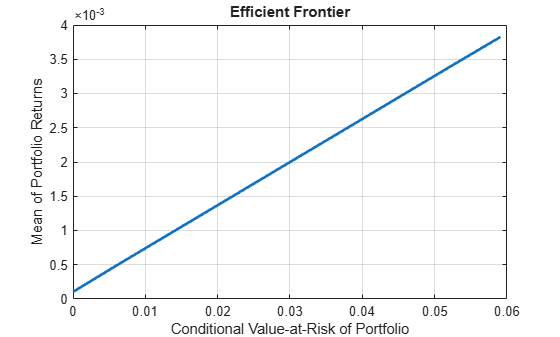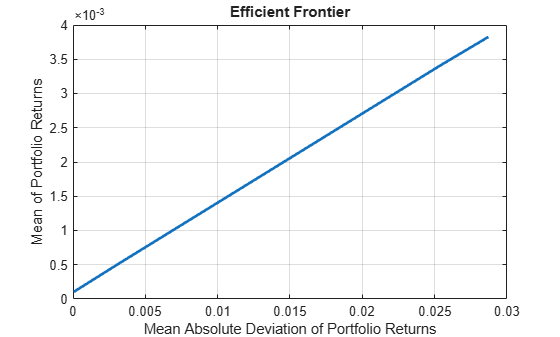# simulateNormalScenariosByData

Simulate multivariate normal asset return scenarios from data

`simulateNormalScenariosByData` has been partially removed and will no longer accept a `fints` object for the `AssetReturns` argument. Use `timetable` instead for financial time series.

Use `fts2timetable` to convert a `fints` object to a `timetable` object.

## Syntax

``obj = simulateNormalScenariosByData(obj,AssetReturns)``
``obj = simulateNormalScenariosByData(obj,AssetReturns,NumScenarios,Name,Value)``

## Description

example

````obj = simulateNormalScenariosByData(obj,AssetReturns)` simulates multivariate normal asset return scenarios from data for portfolio object for `PortfolioCVaR` or `PortfolioMAD` objects. For details on the workflows, see PortfolioCVaR Object Workflow, and PortfolioMAD Object Workflow.```

example

````obj = simulateNormalScenariosByData(obj,AssetReturns,NumScenarios,Name,Value)` simulates multivariate normal asset return scenarios from data for portfolio object for `PortfolioCVaR` or `PortfolioMAD` objects using additional options specified by one or more `Name,Value` pair arguments.This function estimates the mean and covariance of asset returns from either price or return data and then uses these estimates to generate the specified number of scenarios with the function `mvnrnd`. Data can be in a `NumSamples`-by-`NumAssets` matrix of `NumSamples` prices or returns at a given periodicity for a collection of `NumAssets` assets, a `table` or a `timetable`. NoteIf you want to use the method multiple times and you want to simulate identical scenarios each time the function is called, precede each function call with `rng`(seed) using a specified integer seed. ```

## Examples

collapse all

Given a PortfolioCVaR object `p`, use the `simulateNormalScenariosByData` function to simulate multivariate normal asset return scenarios from data.

```m = [ 0.05; 0.1; 0.12; 0.18 ]; C = [ 0.0064 0.00408 0.00192 0; 0.00408 0.0289 0.0204 0.0119; 0.00192 0.0204 0.0576 0.0336; 0 0.0119 0.0336 0.1225 ]; m = m/12; C = C/12; RawData = mvnrnd(m, C, 240); NumScenarios = 2000; p = PortfolioCVaR; p = simulateNormalScenariosByData(p, RawData, NumScenarios)```
```p = PortfolioCVaR with properties: BuyCost: [] SellCost: [] RiskFreeRate: [] ProbabilityLevel: [] Turnover: [] BuyTurnover: [] SellTurnover: [] NumScenarios: 2000 Name: [] NumAssets: 4 AssetList: [] InitPort: [] AInequality: [] bInequality: [] AEquality: [] bEquality: [] LowerBound: [] UpperBound: [] LowerBudget: [] UpperBudget: [] GroupMatrix: [] LowerGroup: [] UpperGroup: [] GroupA: [] GroupB: [] LowerRatio: [] UpperRatio: [] MinNumAssets: [] MaxNumAssets: [] BoundType: [] ```
```p = setDefaultConstraints(p); p = setProbabilityLevel(p, 0.9); disp(p);```
``` PortfolioCVaR with properties: BuyCost: [] SellCost: [] RiskFreeRate: [] ProbabilityLevel: 0.9000 Turnover: [] BuyTurnover: [] SellTurnover: [] NumScenarios: 2000 Name: [] NumAssets: 4 AssetList: [] InitPort: [] AInequality: [] bInequality: [] AEquality: [] bEquality: [] LowerBound: [4x1 double] UpperBound: [] LowerBudget: 1 UpperBudget: 1 GroupMatrix: [] LowerGroup: [] UpperGroup: [] GroupA: [] GroupB: [] LowerRatio: [] UpperRatio: [] MinNumAssets: [] MaxNumAssets: [] BoundType: [4x1 categorical] ```

Create a PortfolioCVaR object `p` and use the `simulateNormalScenariosByData` function with market data loaded from `CAPMuniverse.mat` to simulate multivariate normal asset return scenarios. The market data, `AssetsTimeTable`, is a `timetable` of asset returns.

```load CAPMuniverse p = PortfolioCVaR('AssetList',Assets); disp(p);```
``` PortfolioCVaR with properties: BuyCost: [] SellCost: [] RiskFreeRate: [] ProbabilityLevel: [] Turnover: [] BuyTurnover: [] SellTurnover: [] NumScenarios: [] Name: [] NumAssets: 14 AssetList: {1x14 cell} InitPort: [] AInequality: [] bInequality: [] AEquality: [] bEquality: [] LowerBound: [] UpperBound: [] LowerBudget: [] UpperBudget: [] GroupMatrix: [] LowerGroup: [] UpperGroup: [] GroupA: [] GroupB: [] LowerRatio: [] UpperRatio: [] MinNumAssets: [] MaxNumAssets: [] BoundType: [] ```

Simulate the scenarios from the timetable data for each of the assets from `CAPMuniverse.mat `and plot the efficient frontier.

```p = simulateNormalScenariosByData(p,AssetsTimeTable,10000,'missingdata',true); p = setDefaultConstraints(p); p = setProbabilityLevel(p, 0.9); plotFrontier(p);```Given a PortfolioMAD object `p`, use the `simulateNormalScenariosByData` function to simulate multivariate normal asset return scenarios from data.

```m = [ 0.05; 0.1; 0.12; 0.18 ]; C = [ 0.0064 0.00408 0.00192 0; 0.00408 0.0289 0.0204 0.0119; 0.00192 0.0204 0.0576 0.0336; 0 0.0119 0.0336 0.1225 ]; m = m/12; C = C/12; RawData = mvnrnd(m, C, 240); NumScenarios = 2000; p = PortfolioMAD; p = simulateNormalScenariosByData(p, RawData, NumScenarios); p = setDefaultConstraints(p); disp(p);```
``` PortfolioMAD with properties: BuyCost: [] SellCost: [] RiskFreeRate: [] Turnover: [] BuyTurnover: [] SellTurnover: [] NumScenarios: 2000 Name: [] NumAssets: 4 AssetList: [] InitPort: [] AInequality: [] bInequality: [] AEquality: [] bEquality: [] LowerBound: [4x1 double] UpperBound: [] LowerBudget: 1 UpperBudget: 1 GroupMatrix: [] LowerGroup: [] UpperGroup: [] GroupA: [] GroupB: [] LowerRatio: [] UpperRatio: [] MinNumAssets: [] MaxNumAssets: [] BoundType: [4x1 categorical] ```

Create a PortfolioMAD object `p` and use the `simulateNormalScenariosByData` function with market data loaded from `CAPMuniverse.mat` to simulate multivariate normal asset return scenarios. The market data, `AssetsTimeTable`, is a `timetable` of asset returns.

```load CAPMuniverse p = PortfolioMAD('AssetList',Assets); disp(p.AssetList');```
``` {'AAPL' } {'AMZN' } {'CSCO' } {'DELL' } {'EBAY' } {'GOOG' } {'HPQ' } {'IBM' } {'INTC' } {'MSFT' } {'ORCL' } {'YHOO' } {'MARKET'} {'CASH' } ```

Simulate the scenarios from the timetable data for each of the assets from `CAPMuniverse.mat `and plot the efficient frontier.

```p = simulateNormalScenariosByData(p,AssetsTimeTable,10000,'missingdata',true); p = setDefaultConstraints(p); plotFrontier(p);```## Input Arguments

collapse all

Object for portfolio, specified using a `PortfolioCVaR` or `PortfolioMAD` object.

For more information on creating a `PortfolioCVaR` or `PortfolioMAD` object, see

Data Types: `object`

Asset data that can be converted into asset returns ([`NumSamples`-by-`NumAssets`] matrix), specified as a matrix, `table`, or `timetable`.

`AssetReturns` data can be:

• `NumSamples`-by-`NumAssets` matrix.

• Table of `NumSamples` prices or returns at a given periodicity for a collection of `NumAssets` assets

• Timetable object with `NumSamples` observations and `NumAssets` time series

Data Types: `double` | `table` | `timetable`

Number of scenarios to simulate, specified as a positive integer.

Data Types: `double`

### Name-Value Pair Arguments

Specify optional comma-separated pairs of `Name,Value` arguments. `Name` is the argument name and `Value` is the corresponding value. `Name` must appear inside quotes. You can specify several name and value pair arguments in any order as `Name1,Value1,...,NameN,ValueN`.

Example: ```p = simulateNormalScenariosByData(p,RawData,NumScenarios,'DataFormat','Returns','MissingData',true,'GetAssetList',true)```

Flag to convert input data as prices into returns, specified as the comma-separated pair consisting of `'DataFormat'` and a character vector with the values:

• `'Returns'` — Data in `AssetReturns` contains asset total returns.

• `'Prices'` — Data in `AssetReturns` contains asset total return prices.

Data Types: `char`

Flag to use ECM algorithm to handle `NaN` values, specified as the comma-separated pair consisting of `'MissingData'` and a logical with a value of `true` or `false`.

• `false` — Do not use ECM algorithm to handle `NaN` values (exclude `NaN` values).

• `true` — Use ECM algorithm to handle `NaN` values.

Data Types: `logical`

Flag indicating which asset names to use for the asset list, specified as the comma-separated pair consisting of `'GetAssetList'` and a logical with a value of `true` or `false`.

• `false` — Do not extract or create asset names.

• `true` — Extract or create asset names from the table or timetable.

If a `table` or `timetable` is passed into this function using the `AssetReturns` argument and the `GetAssetList` flag is `true`, the column names from the `table` or `timetable` are used as asset names in `obj.AssetList`.

If a matrix is passed and the `GetAssetList` flag is `true`, default asset names are created based on the `AbstractPortfolio` property `defaultforAssetList`, which is `'Asset'`.

If the `GetAssetList` flag is `false`, no action occurs, which is the default behavior.

Data Types: `logical`

## Output Arguments

collapse all

Updated portfolio object, returned as a `PortfolioCVaR` or `PortfolioMAD` object. For more information on creating a portfolio object, see

## Tips

You can also use dot notation to simulate multivariate normal asset return scenarios from data for a PortfolioCVaR or PortfolioMAD object.

`obj = obj.simulateNormalScenariosByData(AssetReturns,NumScenarios,Name,Value);`